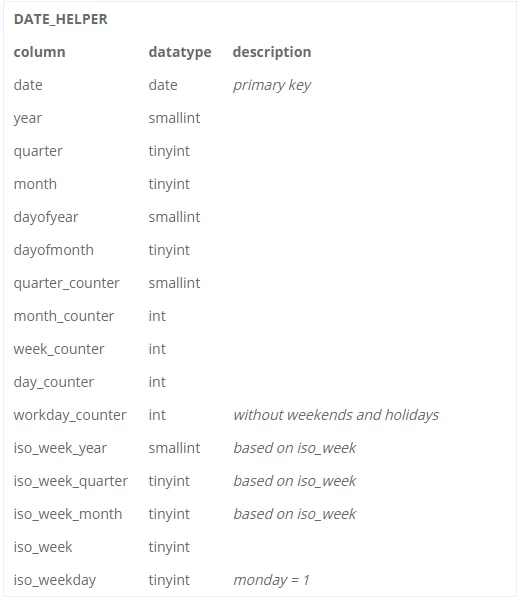# Working with dates in SQL

• 325 viewsUserlevel 6+9
• 546 replies

SQL Server, Oracle and DB2 for IBM i all provide built-in functions to work with dates and times, for instance to calculate the difference between dates, extract specific parts of a date or add/subtract a period from a date. However, using those functions can dramatically decrease the performance of your queries. And when it comes to more advanced date manipulation, e.g. to calculate the number of workdays between two dates or when working with ISO weeks, the built-in functions often fall short. The outcome may even depend on some obscure setting.

This is where a date helper table can help. By creating a static table with additional information about dates you can simply use this table in your queries instead. This blog post provides a sample date helper table that you can adapt to your needs and use in your projects.

## Date helper table

First, create the table using the following definition:Now let’s populate this table using a recursive Common Table Expression. You can put this script in a stored procedure or in the MANUAL code group, to have it executed automatically when creating or upgrading the database.

N.B. This code assumes the existence of a holiday table, containing all holiday dates.

``--Fill the table; with d ([date], workday_counter, iso_weekday)as (    select        convert(date, '1900-01-01'),        1,        1 -- monday    union all    select        dateadd(day, 1, d.[date]),        case            when iso_weekday in (5, 6) then d.workday_counter --weekend            when exists ( --holiday                select 1                 from holiday h                 where h.[date] = dateadd(day, 1, d.[date]))                     then d.workday_counter             else d.workday_counter + 1        end,        iso_weekday % 7 + 1    from d    where d.[date] < convert(date, '2100-01-01'))insert into date_helperselect    d.[date],    datepart(year, d.[date]) as [year],    datepart(quarter, d.[date]) as [quarter],    datepart(month, d.[date]) as [month],    datepart(dayofyear, d.[date]) as [dayofyear],    datepart(day, d.[date]) as [dayofmonth],    datediff(quarter, '1900-01-01', d.[date]) + 1 as quarter_counter,    datediff(month, '1900-01-01', d.[date]) + 1 as month_counter,    datediff(day, '1900-01-01', d.[date]) / 7 + 1 as week_counter,     datediff(day, '1900-01-01', d.[date]) + 1 as day_counter,    d.workday_counter,     null as iso_week_year, --calculated below    null as iso_week_quarter,     null as iso_week_month,     datepart(iso_week, d.[date]) as [iso_week],    d.iso_weekdayfrom doption (maxrecursion 0)--Update ISO stuffupdate hset    iso_week_year = t.[year],    iso_week_quarter = t.[quarter],    iso_week_month = t.[month] from date_helper hjoin date_helper t --thursday  on t.week_counter = h.week_counter  and t.iso_weekday = 4``

Now make sure to create indexes for the fields you filter on, including the fields you often use as non-key (included) columns, and you’re done!

## Sample usage

Calculate the number of workdays between two dates:

``select b.workday_counter - a.workday_counter as workdaysfrom date_helper a, date_helper bwhere a.[date] = '2016-01-01'  and b.[date] = '2016-07-19'``

Retrieve all days from last month:

``select b.[date]from date_helper a, date_helper bwhere a.[date] = '2016-07-19'  and b.month_counter = a.month_counter - 1``

Select ISO date parts, useful for pivot tables or charts:

``select [date], iso_week_year, iso_week_quarter, iso_week_month, iso_week, iso_weekdayfrom date_helperwhere [date] = '2016-01-01'``

Userlevel 2+1

I’ve implemented a solution using the date helper with an extra dimension per week, which had to do with schedules alternating every week, I called this the ‘parity’ of the week.

I wanted the following:

Week parity
51 0
52 1
53 0
01 1
02 0

It seems quite simple at first, just use even and odd weeks, but when you have to account for an optional week 53 the parity will not transition smoothly into the next year, you will get the following:

So I used the latter part or the template (under `Update ISO stuff"`)

``week_parity = t.day_counter %2``
You can simply use different number when you have to account for different situations, for instance when using a 4 week cycle, you simply use `week_parity = t.day_counter %4`